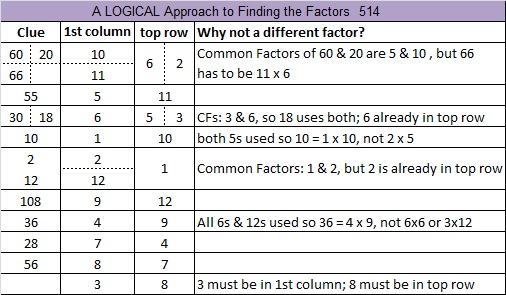514 and Level 5

A rhyme to help kids remember the even digits:

“0-2-4-6-8 being even is just great!”

That rhyme is often followed by another one to help kids remember the odd digits:

1-3-5-7-9 being odd is just fine!

Here’s a fun fact about the number 514:

514^3 = 135796744. Stetson.edu tells us that 514 is the smallest number whose cube starts with all five of those odd digits in consecutive order.

514 is also the hypotenuse of the Pythagorean triple 64-510-514. What is the greatest common factor of those three numbers?Print the puzzles or type the solution on this excel file: 12 Factors 2015-06-01

—————————————————————————————————

• 514 is a composite number.
• Prime factorization: 514 = 2 x 257
• The exponents in the prime factorization are 1 and 1. Adding one to each and multiplying we get (1 + 1)(1 + 1) = 2 x 2 = 4. Therefore 514 has exactly 4 factors.
• Factors of 514: 1, 2, 257, 514
• Factor pairs: 514 = 1 x 514 or 2 x 257
• 514 has no square factors that allow its square root to be simplified. √514 ≈ 22.671568—————————————————————————————————